# Data modeling by least squares

The reconciliation of theory and data is the essence of science. An ubiquitous tool in this task is the method of least-squares fitting. Elementary calculus books generally consider the fitting of a straight line to scattered data points. Such an elementary application gives scant hint of the variety of practical problems which can be solved by the method of least squares. Some geophysical examples which we will consider include locating earthquakes, analyzing tides, expanding the earth's gravity and magnetic fields in spherical harmonics, and doing interesting things with time series. When the past of a time series is available, one may find that least squares can be used to determine a filter which predicts some future values of the time series. When a time series which has been highly predictable for a long stretch of time suddenly becomes much less predictable an event'' is said to have occurred. A filter which emphasizes such events is called a prediction-error filter. If one is searching for a particular dispersed wavelet in a time series, it may help to design a filter which compresses the wavelet into some more recognizable shape, an impulse for example. Such a wave-shaping filter may be designed by least squares. With multiple time series which arise from several sensors detecting waves in space, least squares may be used to find filters which respond only to certain directions and wave speeds.

Before we begin with the general theory, let us take up a simple example in the subject of time series analysis. Given the input, say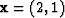to some filter, say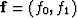then the output is necessarily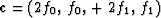. To design an inverse filter we would wish to have c come out as close as possible to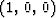. In order to minimize the difference between the actual and the desired outputs we minimize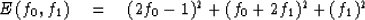The sum E of the squared errors will attain a minimum if f0 and f1 are chosen so that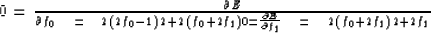Canceling a 2 and arranging this into the standard form for simultaneous equations, we get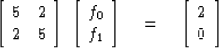and the solution is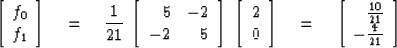The actualwhich comes out of this filter is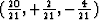which is not a bad approximation to (1, 0, 0).